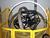## How to Test for Numerics in AS/400Tags:
AS/400
Numeric
RPG ILE
RPG/400
I need to test for Numeric. I have to check, If a variable contains Numeric values. I wanted to use TESTN opcode, but people are saying not to use it; as it does not work properly some times. Is there any other alternative to this? Has anybody done this before? Any help would be of great help. Thanks very much! Svanky

Software/Hardware used:
as/400, rpg/400, rpgle, db2/400, os/400

Thanks. We'll let you know when a new response is added.

You can put a monitor around the %dec function as so:

```Monitor;
On-Error;
//Do whatever you want if field is not numeric
ENDMON;```

This will attempt to convert the string to numeric and catch the error if the field does not contain valid numeric data.

## Discuss This Question: 8 Replies

Thanks. We'll let you know when a new response is added.
•Hi, Here is a way and I think you will receive some more solutions:
```     D IsNumbers       s               n
D Number            s             31s 0
D xDigits               c                        const('0123456789')
/Free
If ( InValue <> ' ' ) and
( %Check(xDigits:%Trim(InValue)) = 0 ) ;
isNumbers = *On ;
Number = %Int(InValue) ;
Else ;
isNumbers = *Off ;
Number = 0 ;
EndIf ;
/End-Free
```
Good luck YuVa47
report
• Svanki, Your question is somewhat unprecise. You write "...check, If a variable contains Numeric values." Several interpretations can be extracted from your question: 1. As the word 'values' i plural your question can be read as 'Are there any continuous strings consisting only of digits 0 to 9 ?' 2. Assuming your question is 'are there any non-numeric values in the variable? 3. How do I test that variable only contains the digits 0 to 9 and that they are continuous and that there are no other characters (except for x'40' (blank)? 4. etc etc Pls clarify (examples pls) your question. DanF
report
•rpg/400, rpgle, db2/400 You linked this with three very different products. You also asked about "a variable" without giving any definition of the variable. An integer variable is always going to be 'numeric'. A zoned decimal variable might or might not be numeric, depending on how you want it to behave. A packed-decimal variable is almost always clearly numeric or not. A character variable may have all numeric digits or possibly be all numeric with blank-padding. Facilities in ILE RPG will be very different from what is provided in DB2 itself. SQL tables will act differently than DDS files. RPG/400 has limitations that aren't present in ILE RPG, whether for file variables or program variables. The way that a program is compiled can change how some non-numeric values are handled. What are example processes that you need to be working within? Tom
report
•Thank You all for your replies. My question is... -------------------- There is a variable with value like this: MyVar = 123X56S89. I need to check individually whether the 1st, 2nd & 3rd bytes contain valid Numeric-value. Based on this(If numeric) I need to do a different process. If not numeric I need to execute a different process altogether. -------------- Pls. note MyVar can contain any value as it is alphanumeric; viz.,12AX56S89, 3WAX57S99, CBAX56S8D, & so on (any value; not restricted to these) Let me know. Thanks
report
•Perhaps something like this .. based on YuVa47 solution Select; // first three are digits when %Check(xDigits:%subst(InValue1,3)) = 0; // do something when %Check(xDigits:%subst(InValue1,2)) = 0; // do something when first two are digits endsl;
report
•OneIsDigit = %Check(xDigits:%subst(InValue:1:1)) = 0; TwoIsDigit = %Check(xDigits:%subst(InValue:2:1)) = 0; ThreeIsDigit = %Check(xDigits:%subst(InValue:3:1)) = 0; Please note, my previous response had the subst wrong .. it needed : between the elements as in this response Phil
report
•Thank You All !! for your replies. What if I use a compile-time array (instead of %CHECK) consisting of 1-element with value '0123456789' and use %LOOKUP of that substringed-byte? (as shown below) Will there be any problem? Iam unable to clearly compare & contrast. pls. let me know ----------------------------------- C Eval Fn1 = %LOOKUP((%SUBST(STRING1:1:1)) : ARRY1) C Eval Fn2 = %LOOKUP((%SUBST(STRING1:2:1)) : ARRY1) C Eval Fn3 = %LOOKUP((%SUBST(STRING1:3:1)) : ARRY1) C IF Fn1 <> 0 and Fn2 <> 0 and Fn3 <> 0 C EVAL FMTTYP = '1' ........ do something ................ C ELSE C EVAL FMTTYP = '2' ........ do something else................ C ENDIF | | **CTDATA NUMB1 0123456789
report
•That will work great. Phil
report